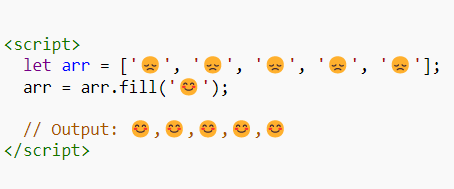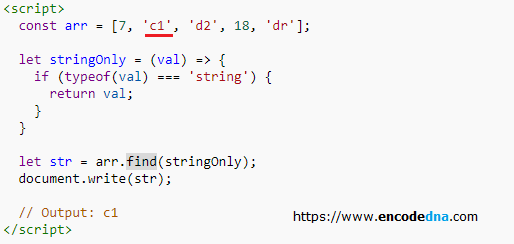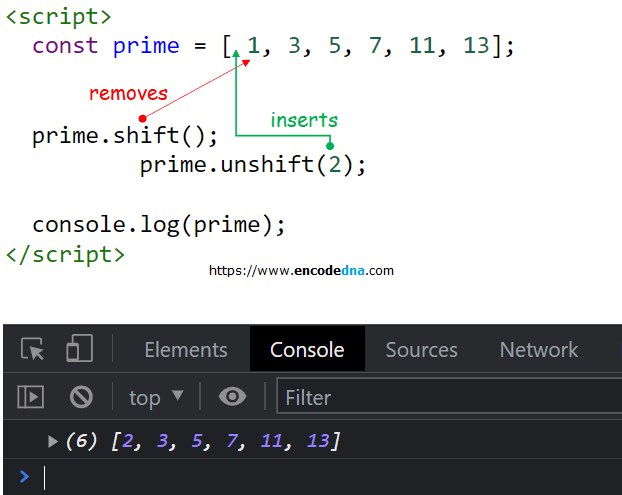﻿ 18 JavaScript Array Methods for Beginners

# 18 JavaScript Array Methods for Beginners

In this article I have shared 18 most important array methods in JavaScript that every beginner should know. If you are a beginner, then you have come to the right place and trust me it will make you job easy. So, let’s get on with it.

## 1) Array.at()

The array.at() takes an integer value, a positive or negative number, as parameter and returns the item at that index in the array. For example,

```<script>
const arr = [2, 4, 6, 8, 10];
document.write(arr.at(3));      // what value is "at" the 3rd index starting from the beginning (left to right).

// Output: 8
</script>```

The .at() method also takes a -ve (negative) value. For example,

```<script>
const arr = [2, 4, 6, 8, 10];
document.write(arr.at(-3));       // Check the 3rd location (or index) starting from the end (right to left).

// Output: 6
</script>```

## 2) Array.every()

The array.every() method checks if all the items in an array are similar or not (or satisfies a given condition).

The method returns a Boolean true or false. So, if all the items meet the condition it returns "true" or it’s a "false". For example,

```<script>
const arrHasEven = [2, 4, 6, 8, 10];
document.write(arrHasEven.every(x => x % 2 === 0));   // Check if array has even numbers.

// Output: true
</script>```

## 3) Array.fill()

You can use the .fill() method to "overwrite" exiting values or items in an array. It can overwrite a specific number of values, from start or end position, or can overwrite the entire array.

The method also takes two parameters.

array.fill(value_to_overwrite, the_index)

Here are two examples. One without the index parameter, and another with the index parameter.

```<script>
let arr = ['😞', '😞', '😞', '😞', '😞'];
arr = arr.fill('😊');		// Fill the array with new values.
document.write(arr);
</script>```

ImageNow, overwrite a specific value at index 2. Not all the values.

```<script>
let arr = ['waiting', 'waiting', 'waiting'];
document.write(arr.fill('booked', 2));  // Here fill() takes 2 parameters. The value to overwrite and at what index.

// Output: waiting,waiting,booked
</script>```

## 4) Array.filter()

The array.filter() method creates a new array from the existing array, based on certain condition.

Its an iterator method. It internally loops through each item in the array to fulfill a given task.

In simple words, this method filters out what you exactly want to extract from the array.

It’s a very powerful and useful method.

Here’s an example.

```<script>
const arr = [7,'c1','d2',18,'dr',21,2];

let numbersOnly = (val) => {
if (typeof(val) === 'number') {
return val;
}
}

let numbers = arr.filter(numbersOnly);	// filter out only numbers from the array.
document.write(numbers);
</script>```

## 5) Array.find()

The array.find() method returns the 1st item in an array that satisfies a particular condition.

For example, I have an array with some numbers and I want to extract the 1st value that is greater than 6. The find() can quickly find and return the value.

```<script>
const arr = [3, 18, 7, 11, 19];
document.write(arr.find(item => item > 6));
</script>```

Now, even there are four numbers that are greater than the value 6, the ".find()" returns the 1st value (number in case) that satisfies the condition. The result is 18.

Here’s another useful example.

```<script>
const arr = [7, 'c1', 'd2', 18, 'dr'];

let stringOnly = (val) => {
if (typeof(val) === 'string') {
return val;
}
}

let str = arr.find(stringOnly);	 // iterates through values, finds and returns the 1st occurrence or match.
document.write(str);

// Output: c1
</script>```

Image## 6) Array.findIndex()

Unlike the find() method that find 1st occurrence of a given value in an array, the array.findIndex() method returns the index of a given value. For example,

```<script>
const arr = ['alpha', 'bravo', 'charlie', 'delta'];
document.write(arr.findIndex(x => x === 'charlie'));	// returns the index of the value. Index start from 0. Therefore, value "charlie" is at index 2.
</script>```

## 7) Array.flat()

The array.flat() method is used to flatten an array. So, if you have a nested array, it flattens the nested array and creates a new array (a single array). You must see the example to understand it better.

```<script>
const arr = ['alpha', 'bravo', ['charlie', 'delta'], 'echo'];
console.log (arr.flat());	// flattens the nested array and creates a new, single array.

// Output: (5) ['alpha', 'bravo', 'charlie', 'delta', 'echo']
</script>```

## 8) Array.flatMap()

The JavaScript array.flatMap() method is a combination of flat() and map() methods. The method first maps each element in an array using the map() method and then flattens it into a new array using the flat() method.

Note: I have explained about the ".flapMap()" method in detail here.

Let’s see the example.

```<script>
const arr = [29, 5, 12, 77, 6];
let arr_flattened = arr.flatMap(
x => x % 2 === 1 ? [x] : []		// return only "odd" numbers.
)

document.write(arr_flattened);

// Output: 29,5,77
</script>```

## 9) Array.forEach()

The forEach() method is one of most important array methods. It is also called an iterator method. You can use this method to call a function once for each element.

forEach() always returns undefined. Therefore, it is not chainable, that is, it cannot be attached with other methods.

For example, I have an array of values (numbers) and want to check if the number is an even number.

```<script>
let getEvenNumbers = (num) => {
if (num % 2 === 0) {
document.writeln(num + "<br />");
}
}

const arr = [12, 6, 11, 9, 2, 62];
arr.forEach(getEvenNumbers);
</script>```

So, the method loops through each value (a number in this case), calls the function "getEvenNumbers()" with value as parameter, the function checks if the value is an even number.

Although, you can do the above using a for loop. However, the "forEach()" is more efficient and quick.

## 10) Array.includes()

I don’t think it is difficult to understand what this method means. The array.includes() method checks if a specified value is in the array. The method returns a Boolean true or false.

```<script>
const arr = ['physics', 'maths', 'sanskrit', 'english', 'computers'];
document.write(arr.includes('sanskrit'));	    // check if subject "sanskrit" includes in the array.
</script>```

## 11) Array.join()

The array.join() method converts an into a string, a single value. It does not change the original array. It just creates a new string using the array elements.

For example, I have an array with numbers and I want to create a new string by joining the numbers, separated by a hyphen (or the "-" minus sign).

```<script>
const arr = [3, 8, 14, 3, 5];
console.log(arr.join('-'));

// Output: 3-8-14-3-5
</script>```

The method takes an argument in the form of separator. In the above example, the separator is hyphen.

If no "separator" is specified, it will return a new string separated by commas. For example,

```<script>
const arr = ['alpha', 'bravo', 'charlie'];
console.log(arr.join());    // join() has no argument. So, default is comma.

// Output: alpha,bravo,charlie
</script>```

## 12) Array.map()

The array.map() method creates a new array by calling a function once for each element in an array.

array.map() is chainable, that is, it can be attached with other methods.

I have explained about the .map() method in detail here.

Now see this example.

```<script>
const arr = [9, 3 , 18, 27, 6];
console.log(arr.map(x => x / 3));	// divide every element by 3.

// Output: (5) [3, 1, 6, 9, 2]
</script>```

Here’s another example.

```<script>
const arr = ['a1', 'c21', 'b9', 'cq87', 'qi8'];

const getOnlyText = (val) => {
return val.match(/\D+/g);
}

document.write(arr.map(getOnlyText));     // It returns only number values from each element.

// Output: a,c,b,cq,qi
</script>```

## 13) Array.pop()

The array.pop() method removes the last element (or item) in an array and returns the element.

```<script>
const arr = [2, 4, 6, 8, 10];
document.write(arr.pop());		// get the last value in the array.
</script>```

Remember, the pop() method creates a new array, minus the last item. See this example.

```<script>
const arr = [2, 4, 6, 8, 10];
console.log(arr.pop());
console.log(arr.pop());

// Output:
10
8
</script>```

It applies to string values also. For example,

```<script>
const arr = ['alpha', 'bravo', 'charlie'];
document.write(arr.pop());

// Output: charlie
</script>```

## 14) Array.push()

The array.push() method is used to add new values to an array. The new value (or item) is added at the end of the array.

```<script>
const arr = ['b1', 'b2', 'b4'];
arr.push('b3');

console.log(arr);

// Output: (4) ['b1', 'b2', 'b4', 'b3']
</script>```

The value "b3" is added at the end of the array.

You can push or add multiple values to the array using this method. For example,

```<script>
const arr = ['b1', 'b2', 'b4'];
arr.push('b3', 'b5', 'b6');
console.log(arr);

// Output: (6) ['b1', 'b2', 'b4', 'b3', 'b5', 'b6']
</script>```

Alternatively, you can use the spread (…) operator to add new items to an array.

## 15) Array.reduce()

The array.reduce() method reduces an array of elements into a single value.

Let’s see an example.

```<script>
const arr = [2, 4, 6, 8, 10];
document.write(arr.reduce ((prev, curr) => prev + curr));		// calculate (addition) all values in the array.

// Output: 30
</script>```

## 16) Array.reverse()

The .reverse() method reverses the order of items in an array. The method however, creates a new array.

```<script>
const arr = [2, 4, 6, 8, 10];
console.log(arr.reverse());     // the method reverses the order of array values

// Output: (5) [10, 8, 6, 4, 2]
</script>```

You can use the ".reverse()" method with other array methods (its chainable). For example,

```<script>
const arr = ['zulu', 'yankee', 'x-ray'];
document.write(arr.reverse().join('<br />'));      // using reverse() and join() together.
</script>```

## 17) Array.shift()

The .shift() method removes the first value in the array and returns the removed value (or item).

It changes the original length of the array. In simple words, the original array is changed when the "shift()" method is used.

```<script>
const arr = [2, 4, 6, 8, 10];
console.log(arr.shift());

// Returns: 2
// The array length: (4) [4, 6, 8, 10]
</script>```

Now, the array is without the 1st item 2, since it has removed it or shifted it. The array items are 4,6,8,10.

## 18) Array.unshift()

The array.unshift() method overwrites the original array by adding one or more items to the beginning of the array. The "length" of array increases, since it will have new items in it.

The .unshift() is the opposite of .push(). The .push() adds new items at the end of an array, the ".unshift()" adds one or more items at the beginning.

```<script>
const arr = [3, 5, 7, 11, 13];
arr.unshift(2);     // You can add multiple values like, arr.unshift(6, 30).
console.log(arr);

// Output: (6) [2, 3, 5, 7, 11, 13]
</script>```

```<script>
const arr = ['car', 'bus'];
arr.unshift('bike');
console.log(arr);

// Output: (3) ['bike', 'car', 'bus']
</script>```

```<script>
const arr = ['eraser', 'printer'];
arr.unshift('pencil', 'keyboard', 'stapler');
console.log(arr);

// Output: (5) ['pencil', 'keyboard', 'stapler', 'eraser', 'printer']
</script>```

## Using ".shift()" and ".unshift()" Methods together

See this array.

```<script>
const prime = [1,3,5,7,11,13];
</script>```

The array above has prime numbers. Oops no. The value "1" is not a prime number. So, at run time I can replace the 1st value using .shift() and add a new value using .unshift() methods

```<script>
const prime = [1,3,5,7,11,13];
prime.shift();
prime.unshift(2);

console.log(prime);    // Output: (6) [2, 3, 5, 7, 11, 13]
</script>```